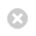Close

Natural Sciences degrees

Home# Mathematics and Statistics

Stream Representative

Aims

While other stream contain some courses in mathematics the Mathematics and Statistics stream offers students the opportunity to give a mathematical flavour to other streams and provides a solid training in quantitative, analytical and computational techniques in their own right.  The skills acquired are generic and complement those provided by other streams in the Natural Sciences programme.

The curriculum focuses on topics that can be applied to a wide variety of scientific disciplines. The mathematics component is concerned with a range of mathematical methods with common applicability in applied mathematics; the statistics component is concerned with the collection and analysis of data to answer questions of substantive interest in the presence of uncertainty.  Both play a fundamental role in the interpretation of information throughout the natural sciences and beyond.

Objectives

Students who complete this stream will gain:

• a sound basis in methods of applied mathematics: differential equations, techniques using complex variables, linear algebra
• an understanding of basic mathematical modelling and problem solving techniques in the field of continuous systems
• skill in the use of a symbolic mathematical computing package for solving problems and mathematical modelling
• an understanding of probability theory and its application to real-world problems
• the ability to use standard techniques to summarise the main features of a set of data, along with basic methods of statistical inference and significance testing in a variety of standard situations
• an understanding of statistical models to answer questions of substantive interest in the presence of uncertainty
Year 1

All Mathematics and Statistics stream students must take:

Year 2

All Mathematics and Statistics stream students must take:

and should choose 15 - 30 credits from:

and may choose 0 - 15 credits from:

Year 3

All Mathematics and Statistics stream students must take:

and may choose 0 - 30 credits from (provided they meet the relevant pre-requisite study):

The Mathematics and Statistics stream is only available as a minor stream and combines with: